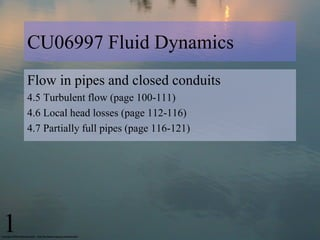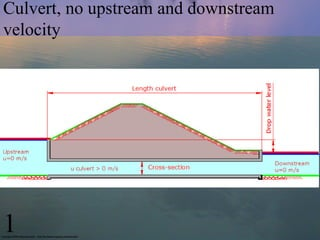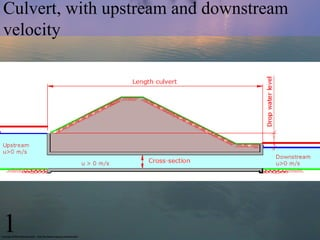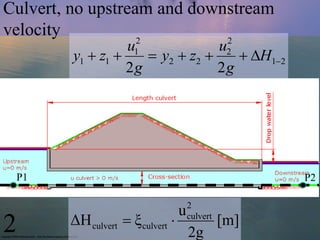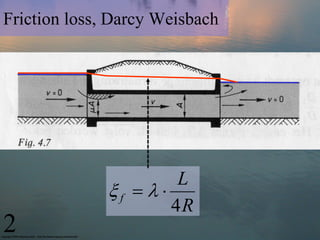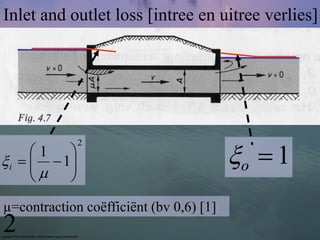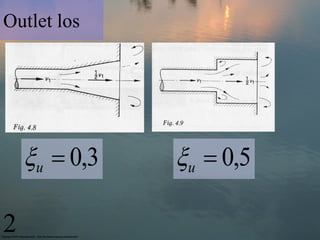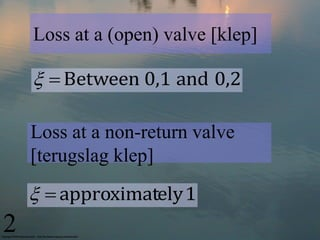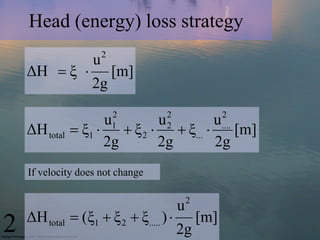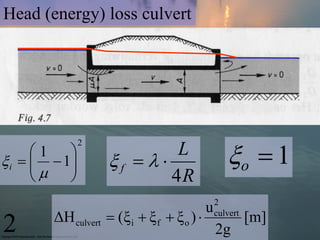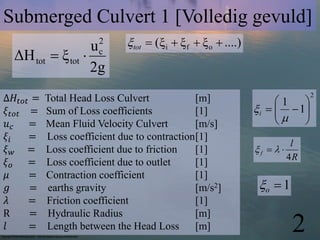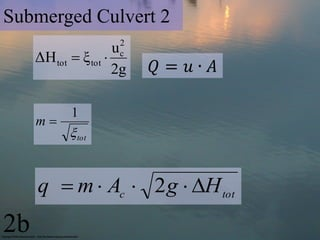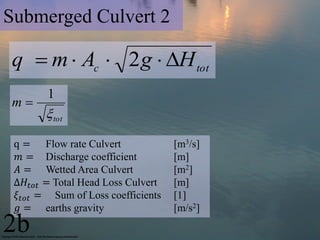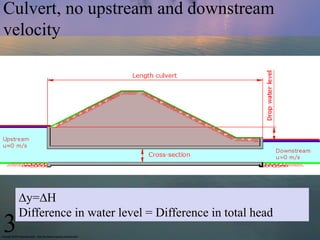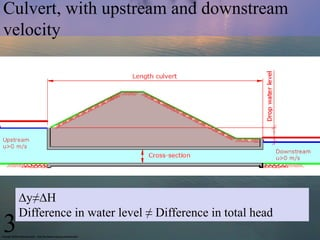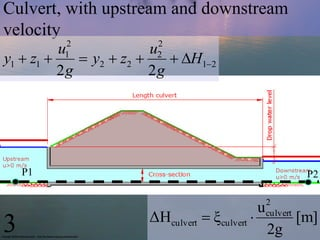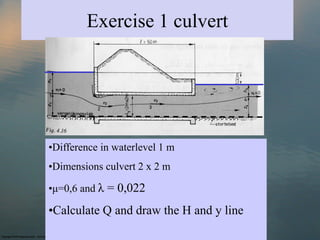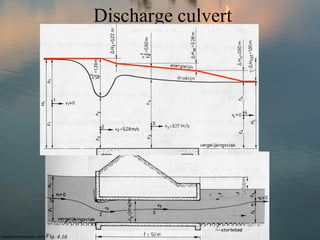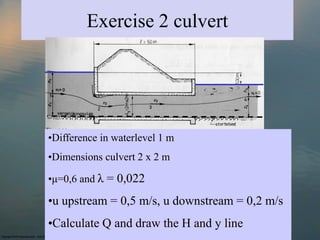1 of 19

### Cu06997 lecture 7_culvert_2013

1. CU06997 Fluid Dynamics Flow in pipes and closed conduits 4.5 Turbulent flow (page 100-111) 4.6 Local head losses (page 112-116) 4.7 Partially full pipes (page 116-121) 1
2. Culvert, no upstream and downstream velocity 1
3. Culvert, with upstream and downstream velocity 1
4. Culvert, no upstream and downstream velocity 2 2 u1 u2 y1  z1   y 2  z2   H12 2g 2g P1 P2 2 u 2 ΔΗ culvert  ξ culvert  culvert [m] 2g
5. Friction loss, Darcy Weisbach L f    4R 2
6. Inlet and outlet loss [intree en uitree verlies] o  1 2 1   i    1     µ=contraction coëfficiënt (bv 0,6)  2
7. Outlet los u  0,3 u  0,5 2
8. Loss at a (open) valve [klep]   Between 0,1 and 0,2 Loss at a non-return valve [terugslag klep]   approximately 1 2
9. Head (energy) loss strategy 2 u ΔΗ  ξ  [m] 2g 2 2 2 u u u ΔΗ total  ξ1   ξ 2   ξ...  1 2 [m] .... 2g 2g 2g If velocity does not change 2 u ΔΗ total  (ξ1  ξ 2  ξ..... )  [m] 2 2g
10. Head (energy) loss culvert o  1 2 1  L  i    1   f      4R 2 u ΔΗ culvert  (ξ i  ξ f  ξ o )  culvert [m] 2 2g
11. Submerged Culvert 1 [Volledig gevuld] u2 tot  (ξ i  ξ f  ξ o  ....) ΔΗ tot  ξ tot  c 2g ∆𝐻 𝑡𝑜𝑡 = Total Head Loss Culvert [m] 1  2 𝜉 𝑡𝑜𝑡 = Sum of Loss coefficients   i    1   𝑢𝑐 = Mean Fluid Velocity Culvert [m/s]   𝜉𝑖 = Loss coefficient due to contraction  l 𝜉𝑤 = Loss coefficient due to friction  f    4R 𝜉𝑜 = Loss coefficient due to outlet  𝜇 = Contraction coefficient  𝑔 = earths gravity [m/s2] o  1 𝜆 = Friction coefficient  R = Hydraulic Radius [m] 𝑙 = Length between the Head Loss [m] 2
12. Submerged Culvert 2 2 u ΔΗ tot  ξ tot  c 2g 𝑄= 𝑢∙ 𝐴 1 m  tot q  m  Ac  2 g  H tot 2b
13. Submerged Culvert 2 q  m  Ac  2 g  H tot 1 m  tot q = Flow rate Culvert [m3/s] 𝑚 = Discharge coefficient [m] 𝐴 = Wetted Area Culvert [m2] ∆𝐻 𝑡𝑜𝑡 = Total Head Loss Culvert [m] 𝜉 𝑡𝑜𝑡 = Sum of Loss coefficients  𝑔 = earths gravity [m/s2] 2b
14. Culvert, no upstream and downstream velocity ∆y=∆H Difference in water level = Difference in total head 3
15. Culvert, with upstream and downstream velocity ∆y≠∆H Difference in water level ≠ Difference in total head 3
16. Culvert, with upstream and downstream velocity 2 2 u u y1  z1  1  y 2  z2  2  H12 2g 2g P1 P2 2 u ΔΗculvert  ξ culvert  culvert [m] 3 2g
17. Exercise 1 culvert •Difference in waterlevel 1 m •Dimensions culvert 2 x 2 m •μ=0,6 and λ = 0,022 •Calculate Q and draw the H and y line
18. Discharge culvert
19. Exercise 2 culvert •Difference in waterlevel 1 m •Dimensions culvert 2 x 2 m •μ=0,6 and λ = 0,022 •u upstream = 0,5 m/s, u downstream = 0,2 m/s •Calculate Q and draw the H and y line